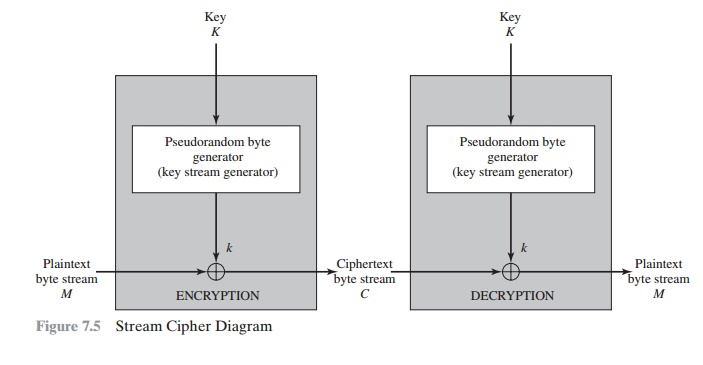# Stream Ciphers

A typical stream cipher encrypts plaintext one byte at a time, although a stream cipher may be designed to operate on one bit at a time or on units larger than a byte at a time.

STREAM CIPHERS

A typical stream cipher encrypts plaintext one byte at a time, although a stream cipher may be designed to operate on one bit at a time or on units larger than a byte at a time. Figure 7.5 is a representative diagram of stream cipher structure. In this structure, a key is input to a pseudorandom bit generator that produces a stream of 8-bit numbers that are apparently random. The output of the generator, called a keystream, is combined one byte at a time with the plaintext stream using the bit- wise exclusive-OR (XOR) operation. For example, if the next byte generated by the generator is 01101100 and the next plaintext byte is 11001100, then the resulting ciphertext byte isDecryption requires the use of the same pseudorandom sequence:The stream cipher is similar to the one-time pad discussed in Chapter 2. The difference is that a one-time pad uses a genuine random number stream, whereas a stream cipher uses a pseudorandom number stream.

[KUMA97] lists the following important design considerations for a stream cipher.

1.        The encryption sequence should have a large period. A pseudorandom num- ber generator uses a function that produces a deterministic stream of bits that eventually repeats. The longer the period of repeat the more difficult it will be to do cryptanalysis. This is essentially the same consideration that was discussed with reference to the Vigenère cipher, namely that the longer the keyword the more difficult the cryptanalysis.

2.        The keystream should approximate the properties of a true random number stream as close as possible. For example, there should be an approximately equal number of 1s and 0s. If the keystream is treated as a stream of bytes, then all of the 256 possible byte values should appear approximately equally often. The more random-appearing the keystream is, the more randomized the ciphertext is, making cryptanalysis more difficult.

3.        Note from Figure 7.5 that the output of the pseudorandom number generator is conditioned on the value of the input key. To guard against brute-force attacks, the key needs to be sufficiently long. The same considerations that apply to block ciphers are valid here. Thus, with current technology, a key length of at least 128 bits is desirable.With a properly designed pseudorandom number generator, a stream cipher can be as secure as a block cipher of comparable key length. A potential advantage of a stream cipher is that stream ciphers that do not use block ciphers as a building block are typically faster and use far less code than do block ciphers. The example in this chapter, RC4, can be implemented in just a few lines of code. Table 7.4, using data from [RESC01], compares execution times of RC4 with three symmetric block ciphers. One advantage of a block cipher is that you can reuse keys. In contrast, if two plaintexts are encrypted with the same key using a stream cipher, then crypt- analysis is often quite simple [DAWS96]. If the two ciphertext streams are XORed together, the result is the XOR of the original plaintexts. If the plaintexts are text strings, credit card numbers, or other byte streams with known properties, then cryptanalysis may be successful.

For applications that require encryption/decryption of a stream of data, such as over a data communications channel or a browser/Web link, a stream cipher might be the better alternative. For applications that deal with blocks of data, such as file transfer, e-mail, and database, block ciphers may be more appropriate. However, either type of cipher can be used in virtually any application.

A stream cipher can be constructed with any cryptographically strong PRNG, such as the ones discussed in Sections 7.2 and 7.3. In the next section, we look at a stream cipher that uses a PRNG designed specifically for the stream cipher.

Study Material, Lecturing Notes, Assignment, Reference, Wiki description explanation, brief detail
Cryptography and Network Security Principles and Practice : One Symmetric Ciphers : Pseudorandom Number Generation and Stream Ciphers : Stream Ciphers |